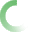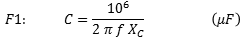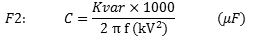﻿ NEPSI - Single-Phase Capacitance CalculatorThe fields with the red border are required.# CALCULATION OF CAPACITANCE

The following calculations can be used to calculate capacitance of a single phase capacitor commonly used on medium and high voltage capacitor banks. Use formula F1 when frequency and the capacitive reactance is are known. Use Formula F2 when the capacitor voltage and kvar and frequency are known.Capacitor frequency (f): Capacitor Reactance (Ohm): Capacitance (uF):Input Capacitor Voltage (kV): Input Capacitor Kvar Rating (kvar): Input Capacitor Frequency Rating (f): Calculated Capacitance (uF): Capacitor Reactance (Ohms):

Table-1 below uses Formula-F1 to calculate the total capacitance when several capacitors are connected in parallel.

 TABLE-1  (Formula-F1) Capacitors Connected in Parallel Capacitor-1 Capacitor-2 Capacitor-3 Capactor-4 Capacitor-5 Capacitor frequency (f): Capacitor Reactance (Ohm): Capacitance (uF): Total Capacitance (uF):

Table-2 below uses Formula F2 to calculate the to capacitance when several capacitors are connected in parallel.

 TABLE-2  (Formula-F2) Capacitors Connected in Parallel Capacitor-1 Capacitor-2 Capacitor-3 Capactor-4 Capacitor-5 Input Capacitor Voltage (kV): Input Capacitor Kvar Rating (kvar): Input Capacitor Frequency Rating (f): Calculated Capacitance (uF): Total Capacitance (uF):